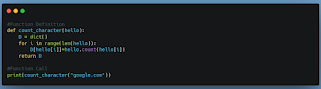# Functions in Python

What is function?
• Function is way of problem solving by dividing the problem into sub-problems and finding their individual solution and combining them to solve original problem.

Function Definition:

• defining the role of function
• actions to be performed by functions are written in the form of statements here.

syntax:

``````    def function_name(parameters_seperated_by_commas):
statements
``````

notice the : and indentation i.e four white spaces.

Some Rules:

• function_name must not be python keyword
• code following colon(:) must be indented.

Function Call:

• calling the defined function
• to execute the work we need to call the defined function.
to execute function, We need to invoke function.

`````` function_name(arguments_seperated_by_commas)
``````

Suppose we want to write a program that draws a house, a car and a cat.
Without use of function we end up writing whole code for drawing house, a car and a cat at a place.

``````     def main():
statements for drawing house
statements for drawing car
statements for drawing cat
``````

which works fine but tracking, debugging is difficult and probably not the best practice.
so what we do here is make function for individual work like:

``````     def draw_house():
statements

def draw_car():
statements

def draw_cat():
statements
``````

and we will call the defined function in our main function as:

``````     def main():
draw_house()
draw_car()
draw_cat()
``

### Types of Functions:

• Void Functions:

• The function that doesn't return anything.
• simply speaking, The return type is None
for example:
If we want to add two numbers given to function and print it's result then:

``````def addition(num1,num2):
print(f"{num1}+{num2}=",num1+num2)
``````
• Fruitful Functions:

• The function that returns some value
• The return type is not null.
for example:

``````def addition(num1,num2):
return num1+num2
``````